# Power in AC Circuits : Instantaneous and Average Power

Average power formula is the important calculation in the electrical circuit.

The instantaneous power p(t) absorbed by an element is the product of the instantaneous voltage v(t) across the element and the instantaneous current i(t) through it.

Make sure to read what is ac circuit first.

## Instantaneous and Average Power Formula

Assuming the passive sign convention,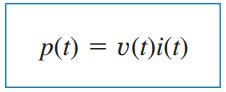(1)

The instantaneous power (in watts) is the power at any instant of time.

It is the rate at which an element absorbs energy.

Consider the general case of instantaneous power absorbed by an arbitrary combination of circuit elements under sinusoidal excitation, as shown in Figure.(1).Figure 1. Sinusoidal source and passive linear circuit

Let the voltage and current at the terminals of the circuit be(2a)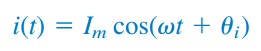(2b)

where Vm and Im are the amplitudes (or peak values), and θv and θi are the phase angles of the voltage and current, respectively.

The instantaneous power absorbed by the circuit is(3)

We apply the trigonometric identity(4)

and express Equation.(3) as(5)

This shows us that the instantaneous power has two parts. The first is constant or time-independent. Its value depends on the phase difference between the voltage and the current.

The second is a sinusoidal function whose frequency is 2ω, which is twice the angular frequency of the voltage or current.

The sketch of p(t) in Equation.(5) is shown in Figure.(2), where T = 2π/ω is the period of voltage or current.

We observe that p(t) is periodic, p(t) = p(t + T0), and has a period of T0 = T/2, since its frequency is twice that of voltage or current.

We also observe that p(t) is positive for some part of each cycle and negative for the rest.

When p(t) is positive, power is absorbed by the circuit.

When p(t) is negative, power is absorbed by the source; that is, power is transferred from the circuit to the source.

This is possible because of the storage elements (capacitors and inductors) in the circuit.Figure 2. The instantaneous power p(t) entering a circuit

The instantaneous power changes with time and is therefore difficult to measure.

The average power is more convenient to measure. In fact, the wattmeter, the instrument for measuring power, responds to average power.

The average power, in watts, is the average of the instantaneous power over one period.

Thus, the average power is given by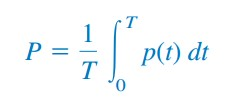(6)

Although Equation.(6) shows the averaging done over T, we would get the same result if we performed the integration over the actual period of p(t) which is T0 = T/2.

Substituting p(t) in Equation.(5) to (6) results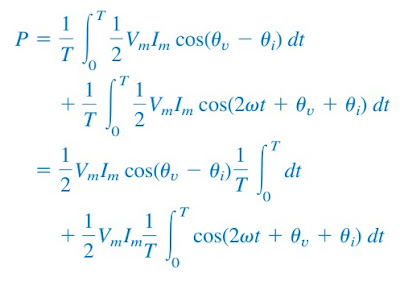(7)

The first integrand is constant, and the average of a constant is the same constant. The second integrand is a sinusoid.

We know that the average of a sinusoid over its period is zero because the area under the sinusoid during a positive half-cycle is cancelled by the area under it during the following negative half-cycle.

Thus, the second term in Equation.(7) vanishes and the average power becomes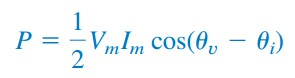(8)

Since cos(θv – θi) = cos(θv – θi), what is important is the difference in the phases of the voltage and current.

Note that p(t) is time-varying while P does not depend on time. To find the instantaneous power, we must necessarily have v(t) and i(t) in the time domain.

But we can find the average power when voltage and current are expressed in the time domain, as in Equation.(8), or when they are expressed in the frequency domain.

The phasor form of v(t) and i(t) in Equation.(2) are V = Vm∠θv and I = Im∠θi, respectively.

P is calculated using Equation.(8) or using phasors V and I. To use phasors, we notice that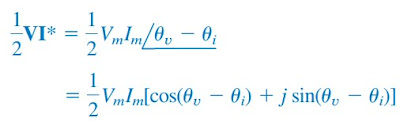(9)

We recognize the real part of this expression as the average power P according to Equation.(8). Hence,(10)

Consider two special cases of Equation.(10). When θvi, the voltage and current are in phase. This implies a purely resistive circuit or resistive load R, and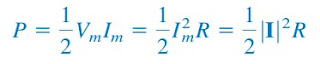(11)

where |I|2 = I x I*. Equation.(11) shows that a purely resistive circuit absorbs power at all times. When θv – θi = ± 90o, we have a purely reactive circuit, and(12)

showing that a purely reactive circuit absorbs no average power. In summary,

A resistive load (R) absorbs power at all times, while a reactive load (L or C) absorbs zero average power.

## Instantaneous and Average Power Formula Examples

For better understanding let us review the examples below
1. Given that
v(t) = 120 cos(377t + 45o) V and i(t) = 10 cos(377t – 10o) A

find the instantaneous power and the average power absorbed by the passive linear network of Figure.(1)

Solution :
The instantaneous power is given by

Applying the trigonometric identity

gives

or

The average power is

which is the constant part of p(t) above.

2. Calculate the average power absorbed by an impedance Z = 30 – j70 Ω when a voltage V = 120 ∠0o is applied across it

Solution :
The current through the impedance is

The average power is

3. For the circuit in Figure.(3), find the average power is supplied by the source and the average power absorbed by the resistor.Figure 3

Solution :
The current I is given by

The average power supplied by the voltage source is

The current through the resistor is

and the voltage across it is

The average power absorbed by the resistor is

which is the same as the average power supplied. Zero average power is absorbed by the capacitor.

4. Determine the average power generated by each source and the average power absorbed by each passive element in the circuit of Figure.(4a).Figure 4

Solution :
We apply to mesh analysis as shown in Figure.(4b). For mesh 1,

For mesh 2,

or

For the voltage source, the current flowing from it is I2 = 10.58∠79.1o A and the voltage across it is 60∠30o V, so that the average power is

Following the passive sign convention, this average power is absorbed by the source, in view of the direction of I2 and the polarity of the voltage source.

That is, the circuit is delivering average power to the voltage source.

For the current source, the current through it is I1 = 4∠0o and the voltage across it is

The average power supplied by the current source is

It is negative according to the passive sign convention, meaning that the current source is supplying power to the circuit.

For the resistor, the current through it is I1 = 4∠0o and the voltage across it is 20I1 = 80∠0o, so that the power absorbed by the resistor is

For the capacitor, the current through it is I2 = 10.58∠79.1o and the voltage across it is –j5I2 = (5∠-90o)(10.58∠79.1o) = 52.9∠79.1 – 90.

The average power absorbed by the capacitor is

For the inductor, the current through it is I1 – I2 = 2 – j10.39 = 10.58∠-79.1o.

The voltage across it is j10(I1 – I2) = 10.58∠-79.1o + 90o . Thus, the average power absorbed by the inductor is

Notice that the inductor and the capacitor absorb zero average power and that the total power supplied by the current source equals the power absorbed by the resistor and the voltage source, or

indicating that power is conserved.

Have you understood what is instantaneous power? Don’t forget to share and subscribe! Happy learning!

Reference:  Fundamentals of electric circuits by Charles K. Alexander and Matthew N. O. Sadiku